# E-PolyLearning

 1. Select the alternative which represents three out of the five alternative figures which when fitted into each other would form a complete square.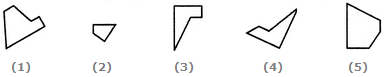a. 145 b. 245 c. 123 d. 234

 2. Select the alternative which represents three out of the five alternative figures which when fitted into each other would form a complete square.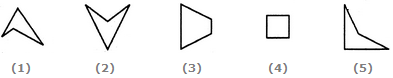a. 124 b. 345 c. 123 d. 135
 3. Select the alternative which represents three out of the five alternative figures which when fitted into each other would form a complete square.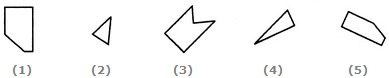a. 124 b. 125 c. 234 d. 245
 4. Select the alternative which represents three out of the five alternative figures which when fitted into each other would form a complete square.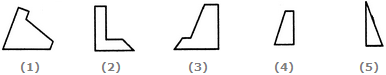a. 123 b. 124 c. 135 d. 145
 5. Select the alternative which represents three out of the five alternative figures which when fitted into each other would form a complete square.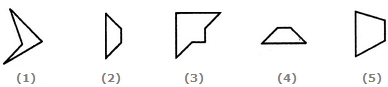a. 134 b. 345 c. 234 d. 135